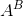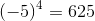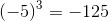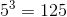GMAT Math : Arithmetic

Example Questions

1 2 24 25 26 27 28 29 30 32 Next →

Example Question #11 : Dsq: Understanding Powers And Rootsandare integers. Ispositive, negative, or zero?

Statement 1:is negative.

Statement 2:is odd.

Both statements TOGETHER are insufficient to answer the question.

Statement 1 ALONE is sufficient to answer the question, but Statement 2 ALONE is not sufficient to answer the question.

Either statement ALONE is sufficient to answer the question.

Statement 2 ALONE is sufficient to answer the question, but Statement 1 ALONE is not sufficient to answer the question.

Both statements TOGETHER are sufficient to answer the question, but neither statement ALONE is sufficient to answer the question.

Both statements TOGETHER are sufficient to answer the question, but neither statement ALONE is sufficient to answer the question.

Explanation:

A negative integer to an even power is positive:

Example:A negative integer to an odd power is negative:

Example:A positive integer to an odd power is positive:

Example:So, as seen in the first two statements, knowing only that baseis negative is insufficient to detemine the sign of; as seen in the last two statements, knowing only that exponentis odd is also insufficient. But by the middle statement, knowing both facts tells usis negative.

The answer is that both statements together are sufficient to answer the question, but neither statement alone is sufficient.

1 2 24 25 26 27 28 29 30 32 Next →

Tired of practice problems?

Try live online GMAT prep today.